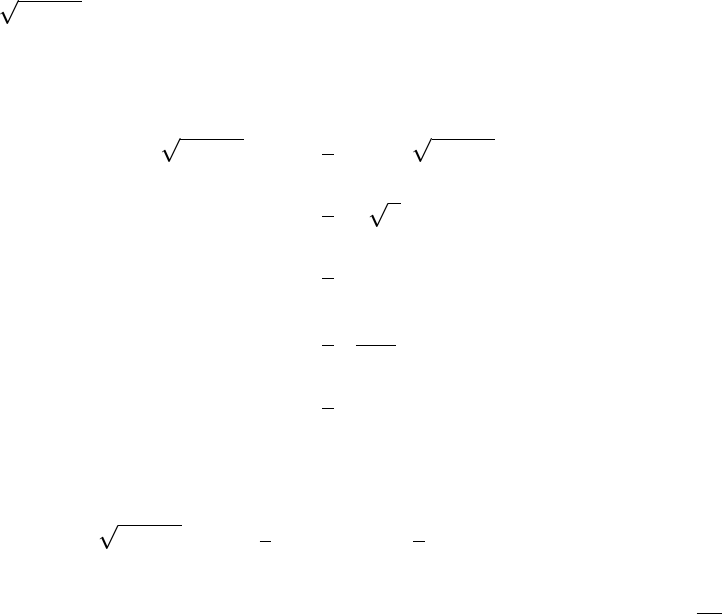Study Guides (400,000)
US (230,000)
NCSU (600)
MA (50)
MA 141 (40)
Final

# MA 141 Lecture 24: SubstitutionExam

Department
Mathematics
Course Code
MA 141
Professor
Kevin Flores
Study Guide
Final

This preview shows pages 1-2. to view the full 6 pages of the document.MA 141 Chapter 4
Section 4.4: Substitution
As we saw previously, sometimes integrals represent a function composition. Substitution is
a method to deal with such integrals. Recall that if H(x) = F(g(x)) and F=f, then
H(x) = F(g(x)) ·g(x) = f(g(x)) ·g(x)
Zf(g(x)) ·g(x)dx =F(g(x)) + C.
We can simplify the problem by letting u=g(x). Recall that we said in Section 3.6 if
y=f(x), then dy =f(x)dx. Then here we have du =g(x)dx and
Zf(g(x)) ·g(x)dx =Zf(u)du =F(u) + C=F(g(x)) + C.
Using this change of variables is called the substitution method. For substitution to work,
we pick part of our integrand to be uand ensure that this relabeling with uand du leaves
behind none of the old variable (the change must be complete).
Ex: Find Zx6x2dx.
Let’s try using u= 6x2. Then du =2xdx. How does this relabel our integral? We cannot
relabel just yet because we do not have the full du term. We are missing the coeﬃcient 2.
Zx6x2dx =1
2Z2x6x2dx
=1
2Zudu
=1
2Zu1/2du
=1
2·u3/2
3/2+C
=1
3u3/2+C
We always want to go back to our original variable. Since u= 6 x2, we have
Zx6x2dx =1
3u3/2+C=1
3(6 x2)3/2+C
Note: we could also solve for x dx and substitute that in to the integral. x dx =du
2.
1

Only pages 1-2 are available for preview. Some parts have been intentionally blurred.Ex: Zx6x2dx.
What happens if we choose something else for u? Could it still work?
du =1
2(6 x2)1/2(2x)dx
=xdx
6x2
6x2du =xdx
u du =x dx
Zx6x2dx =Zu(u du)
=Zu2du
=1
3u3+C
=1
3(6 x2)3/2+C
Ex: Find Zsin(x) cos(x)dx.
What should I choose for u? Try u= sin(x),du = cos(x)dx.
Zsin(x) cos(x)dx =Zu du
=u2
2+C
=sin2x
2+C
Can I use u= cos x? Then du =sin xdx.
Zsin(x) cos(x)dx =Zcos x(sin(x))dx
=Zu du
=u2
2+C
=cos2x
2+C